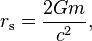# Observable universe as a black hole

A substance of arbitrarily low density can form a black hole if there is enough of it.

So I took the mean density of the universe and calculated how big it would have to be to form a black hole. It's a surprising coincidence that the swartzchild radius in light years is the exact age of the universe. Is this just a coincidence or is the universe analogous to a black hole?

PeterDonis
Mentor
2020 Award
I took the mean density of the universe and calculated how big it would have to be to form a black hole.

How did you do that calculation? A black hole doesn't have a volume in the ordinary sense, so it doesn't have a density in the ordinary sense either.

How did you do that calculation? A black hole doesn't have a volume in the ordinary sense, so it doesn't have a density in the ordinary sense either.

I used the formula:With m (of universe)= 10^53 kg
This gives 15.6 light years as the swartzchild radius. Which is pretty close to the age of the universe.

I was also thinking that the matter escaping our cosmological event horizon might be analogous to a black hole losing mass via Hawking radiation, with the rate of expansion increasing as the rate of evaporation increases.

phinds
Gold Member
This gives 15.6 light years as the swartzchild radius. Which is pretty close to the age of the universe.
You might want to give some thought to the units involved here. Your statement is comparing apples (distance) and oranges (duration).

FURTHER, 15billion years (give or take) is not the distance from which the light now reaching us was emitted (that was much less than 15 billion light years), nor is it the distance the the emitting objects are now (that's more like 47 billion light years)

PeterDonis
Mentor
2020 Award
This gives 15.6 light years as the swartzchild radius.

I think you mean 15.6 billion light-years, correct?

Which is pretty close to the age of the universe.

First of all, this has nothing to do with "taking the mean density of the universe and calculating how big it would have to be to form a black hole", which is what you described in your OP. You're just calculating the horizon radius of a black hole with the mass of the observable universe. As I said before, a black hole doesn't have a "volume" in the ordinary sense, so calculating its horizon radius doesn't tell you anything about how much space is inside the horizon. (In fact, that question doesn't even have a well-defined answer.)

Second, if you were going to somehow model the observable universe as a black hole, the key point is not its age, but the surface area of the sphere that encloses it. Not only does this avoid any mismatch of units (as phinds points out), it also takes into account what I said above, that a black hole doesn't have a well-defined interior volume. What you should really be comparing is the surface area of the black hole in question, which is just ##4 \pi## times the radius you calculated (15.6 light-years) squared, with the surface area of a sphere enclosing the observable universe.

Unfortunately, this comparison is not "pretty close". According to our best current model, the observable universe is spatially flat, and has a current radius of about 47 billion light-years, so a sphere enclosing it would have a surface area of ##4 \pi## times 47 billion squared. This is quite a bit bigger than ##4 \pi## times 15.6 billion squared.

(There is also the point that the universe, unlike a black hole's horizon, is not static--it is expanding; but going into that would add even more complications, and I've already said enough to show why the idea of the observable universe being a black hole is not tenable.)

Ok thanks for that guys.